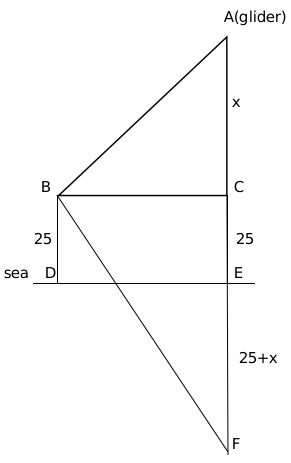Question 3

# The angle of elevation of a glider from the deck of ship 25 meters above the sea surface is $$30^\circ$$ nd the angle of depression of the reflection of glider in the sea is $$75^\circ$$. Find the approximate height of the glider from the sea surface. $$\sqrt{3}=\frac{19}{11}$$

SolutionIt is given, angle ABC = 30 degrees and angle FBC = 75 degrees

$$\tan\ 30^{\circ\ }=\frac{x}{BC}$$

$$BC\ =\ x\sqrt{\ 3}$$

$$\tan75^{\circ\ }=\ \frac{\ 50+x}{x\sqrt{\ 3}}$$

$$2+\sqrt{\ 3}\ =\ \frac{\ 50+x}{x\sqrt{\ 3}}$$

Solving we get x = 9.1

Height of glinder from sea surface = 25+x $$\approx\$$ 34